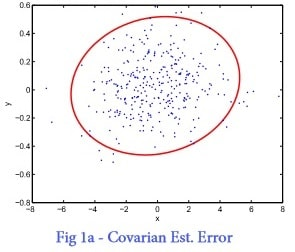# Linear Systems Homework Assignment 6

Problem 1

Figure 1-left a Monte Carlo simulation of a random variable and its error ellipse.

1. From the error ellipse shown in Figure 1a, what is the mean value? Give an approximate value.
2. Plot the data points and the error ellipse knowing that the mean value of a random Guassian variable is

µx = [12,33]T (1)

and its covariance is Cx = [1 2; 1 1] (2)

You can use Matlab function randn of other functions. Questions 1) and 2) do not necessarily refer to the same variable.

3. Another variable is define as y = Ax (3)
4. Plot the data points and the error ellipse of y. Is y Gaussian?
5. Find the variance and mean of y using the data points.
6. Find the variance and mean of y using the error propagation equation. Compare with previous question.

## PLOT THIS FOR PROBLEM #1Fig. 1. Covariance estimation error and Kalman filtering